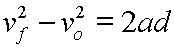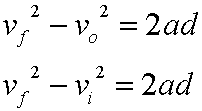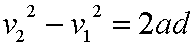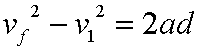Here is our time independent equation:There are other popular ways to write the symbols in this equation.

(What follows here, basically, is the same type of discussion that appears in the corresponding section for the velocity equation. An additional note, though, appears here after this section.)

There is more that one symbol for the original velocity. All three of these symbols cover the same concept:

 vo Original velocity vi Initial velocity v1 First velocity

And there is more that one symbol for the final velocity. Both of these symbols mean the same thing:

 vf Final velocity v2 Second velocity

Basically, these velocity symbols fall into two families. These go together in one family:

vo, vi, and vf

These go together in another family:

v1 and v2

So, using the first family of velocity symbols, note that these two equations mean the same thing:Using the second set of symbols, again, we say the same idea with this notation:When you write the equation you would never mix a symbol from one family with a symbol from another. So, you would not write something like this:Of course, since this equation is time independent, you can not think of it as a function of time as you could for our other two equations.

Custom Search